Courses

# RS Aggarwal Test: Statistics

## 25 Questions MCQ Test Mathematics (Maths) Class 10 | RS Aggarwal Test: Statistics

Description
This mock test of RS Aggarwal Test: Statistics for Class 10 helps you for every Class 10 entrance exam. This contains 25 Multiple Choice Questions for Class 10 RS Aggarwal Test: Statistics (mcq) to study with solutions a complete question bank. The solved questions answers in this RS Aggarwal Test: Statistics quiz give you a good mix of easy questions and tough questions. Class 10 students definitely take this RS Aggarwal Test: Statistics exercise for a better result in the exam. You can find other RS Aggarwal Test: Statistics extra questions, long questions & short questions for Class 10 on EduRev as well by searching above.
QUESTION: 1

### Which of the following is a measure of central tendency?

Solution:

In statistics, a central tendency is a central or typical value for a probability distribution. It may also be called a center or location of the distribution. Colloquially, measures of central tendency are often called averages. So we have 3 measures of central tendencies namely, mean , median and mode. So mean is the answer as the other three options are the components of the given distribution.

QUESTION: 2

### Class mark of a class is obtained by using –

Solution:

Class mark is the mean of the upper limit and lower limit of the class. The class mark is a specific point in the center of the interval in a frequency distribution table. It is defined as the average of the upper and lower class limits. So formula is Class mark =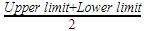QUESTION: 3

### The value of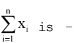Solution:

We have Mean =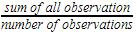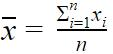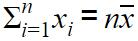QUESTION: 4

The mean of the following data 12, 22, 32,.......n2 is –

Solution:
QUESTION: 5

The mean of following distribution is –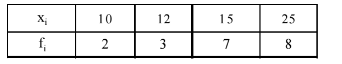Solution:
QUESTION: 6

The mean of the following data is 18.75 then the value of p is –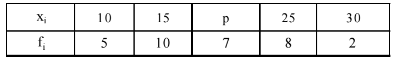Solution:
QUESTION: 7

To find mean, we use the formula.

Solution:
QUESTION: 8

Which of the following can not be determined graphically –

Solution:

Mode is the value with the maximum frequency. Thus, it can be determined from the graph.
Median is the middle value of the data. Thus, it can be determined from the graph.
Mean is the ratio of sum of all data values and the total number of values. Thus, it cannot be determined graphically.
The bell curve or standard deviation graph is used to visualise the spread of data. The standard deviation tells how much the data is clustered around the mean of the data. Lower standard deviation tells that the data is less spread and higher standard deviation tells that data is more spread.

QUESTION: 9

If the median of the following data is 40 then the value of p is –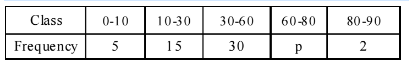Solution:
QUESTION: 10

Which of the following is true?

Solution:
QUESTION: 11

Mode is –

Solution:
QUESTION: 12

Which of the following is true –

Solution:

Mean = Mode + 3/2 [Median – Mode]
Mean= Mode+3/2 Median-3/2Mode
2 Mean=2 Mode+3 Median-3 Mode
3 Median=2 Mean + Mode
So C is the correct answer.

QUESTION: 13

In the formula for mode of a grouped data, mode =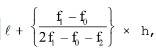where symbols have their usual meaning f0 represents :

Solution:

f1 is the frequency of the modal class.So , fis the frequency of the class preceding the modal class. So C option is correct.

QUESTION: 14

Median of a given frequency distribution is found with the help of a –

Solution:
QUESTION: 15

The measure of central tendency which is given by the x-coordinate of the point of intersection of the 'more than' ogive and 'less than' ogive is –

Solution:
QUESTION: 16

The mean of the first 10 natural numbers is

Solution:

The first 10 natural numbers are 1, 2, 3, 4, 5, 6, 7, 8, 9 and 10.

Mean = Sum of Observations/Total No of Observations

Mean = 1 + 2 + 3 + 4 + 5 + 6 + 7 + 8 + 9 + 10 ÷ 10

Mean = 55/10

Mean = 5.5

QUESTION: 17

The mean of the first 10 prime numbers is

Solution:

Step-by-step explanation:mean=2+3+5+7+11+13+17+19+23+29/10

Mean=129/10

Mean=12.9

QUESTION: 18

The mean of first 10 composite numbers is

Solution:
QUESTION: 19

The marks obtained by 9 students in Mathematics are 59, 46, 31, 23, 27, 40, 52, 35 and 29. The mean of the data is

Solution:

The mean is the average of the numbers.
So, Mean =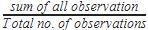Mean=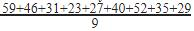=342/9=38

QUESTION: 20

The mean of the first 10 natural odd numbers is

Solution:

Natural numbers start from 1
First ten natural odd numbers=1,3,5,7,9,11,13,15,17,19
Mean =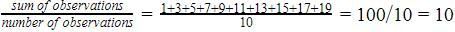So mean is 10.

QUESTION: 21

The mean of the first 10 multiples of 6 is

Solution:

We have first ten multiples of 6 as follows:
6,12,18,24,30,36,42,48,54,60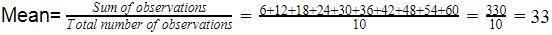QUESTION: 22

The mean of all the factors of 24 is

Solution:
QUESTION: 23

The marks obtained by 9 students in Mathematics are 59, 46, 31, 23, 27, 44, 52, 40 and 29. The mean of the data is

Solution:

The mean is the average of the numbers.
So, Mean =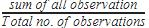Mean=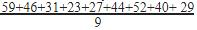351/9=39

QUESTION: 24

The arithmetic mean of a set of 40 values is 65. If each of the 40 values is increased by 5, what will be the mean of the set of new values:

Solution:

We have mean =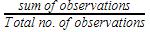Mean =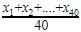Now each value is increased by 5
Mean =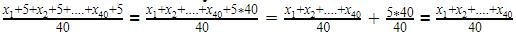+5=65+5=70
Hence the mean is 70

QUESTION: 25

The mean of 25 observations is 36. If the mean of first 13 observations is 32 and that of the last 13 observations is 39, then the 13th observation is

Solution:
Mean of 25 observation = 36
Sum of 25 observations = 36 x 25 = 900

Mean of first 13 observations = 32
Sum of first 13 observations = 32 x 13 = 416

Mean of last 13 observations = 39
Sum of last 13 observations= 39 x 13 = 507

13th observation = (416 + 507) - 900
13th observation= 923 - 900
So, 13th observation = 23